Page No. 1034

Math / Math Worksheets / Halloween Worksheets Printables

# 🎃 Free Math Halloween Worksheets PDF

Our free math Halloween worksheets for kids were created for use at home or in the classroom.

Download printable Halloween maths activities in PDF for this super exciting time of year and channel your students' enthusiasm for Halloween into fun learning experiences.

Here are your free Halloween math worksheets in PDF.

## 👻 Free math Halloween worksheets for kids

Generate free Halloween math worksheets in PDF. Our free printable math Halloween worksheets help kids to enjoy Halloween celebration and to learn math at the same time. You can also solve these Halloween math puzzles online here.

## Halloween math worksheets FREE

These Halloween math puzzles are generated automatically.

Just click on the image and a new Halloween math worksheet in PDF will be created.

These Halloween worksheets are generated in 3 levels, they contain addition, subtraction and multiplication problems. And of course, these Halloween math sheets contain also the answer key on the second page.

This Halloween game challenges students to use their math and reasoning skills to find the value of 3 different symbols contained within a puzzle.

Most of these fun Halloween worksheets for kids are generated automatically, so every time you get a new Halloween math sheet in PDF. So, if there is written ´Generate a new PDF´ under the worksheet, you can download as many different free Halloween activities in PDF as you want.

### Number matching

Generate number matching worksheets 1-10.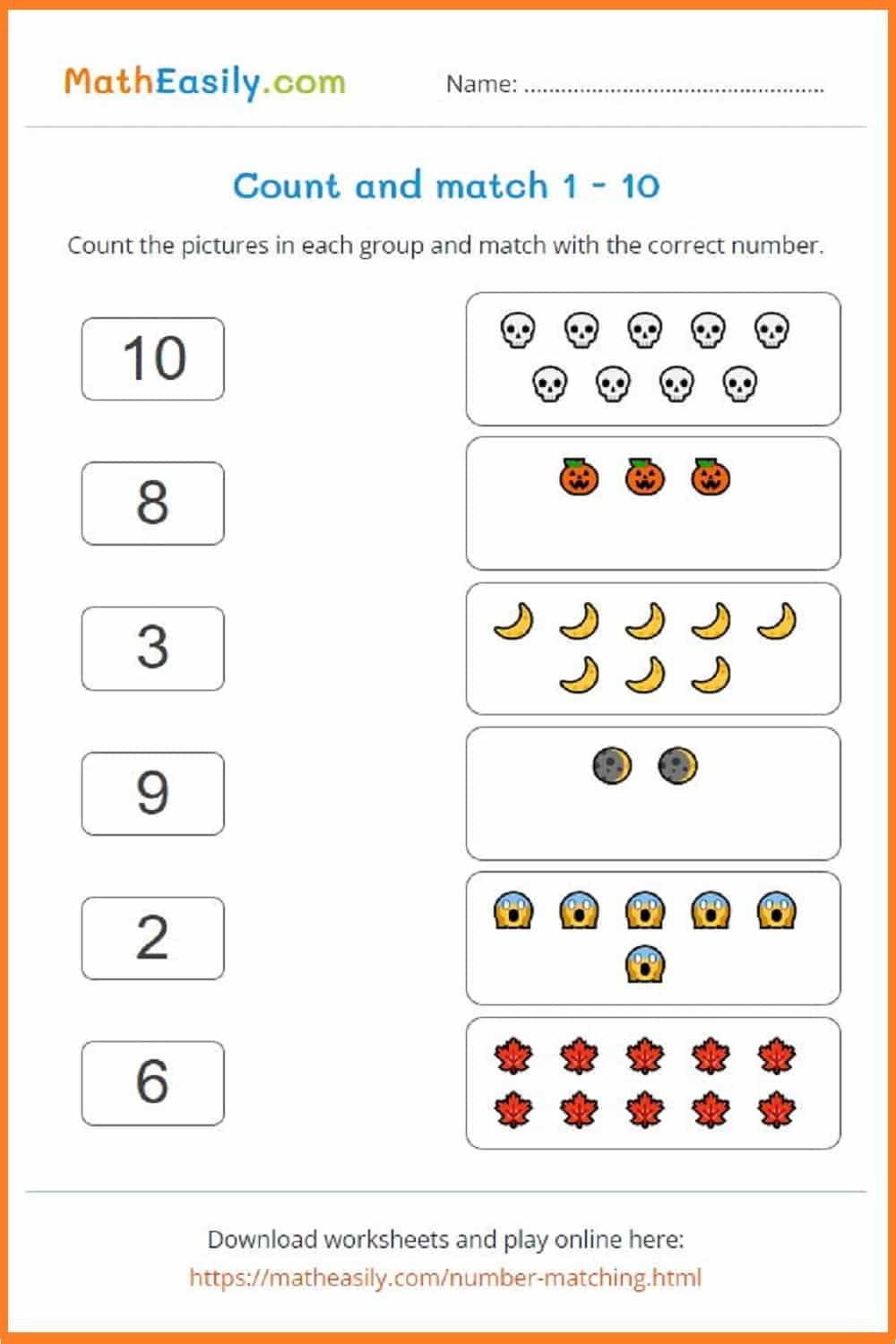Generate a new PDF

### Number matching

Generate number matching puzzles 1-20.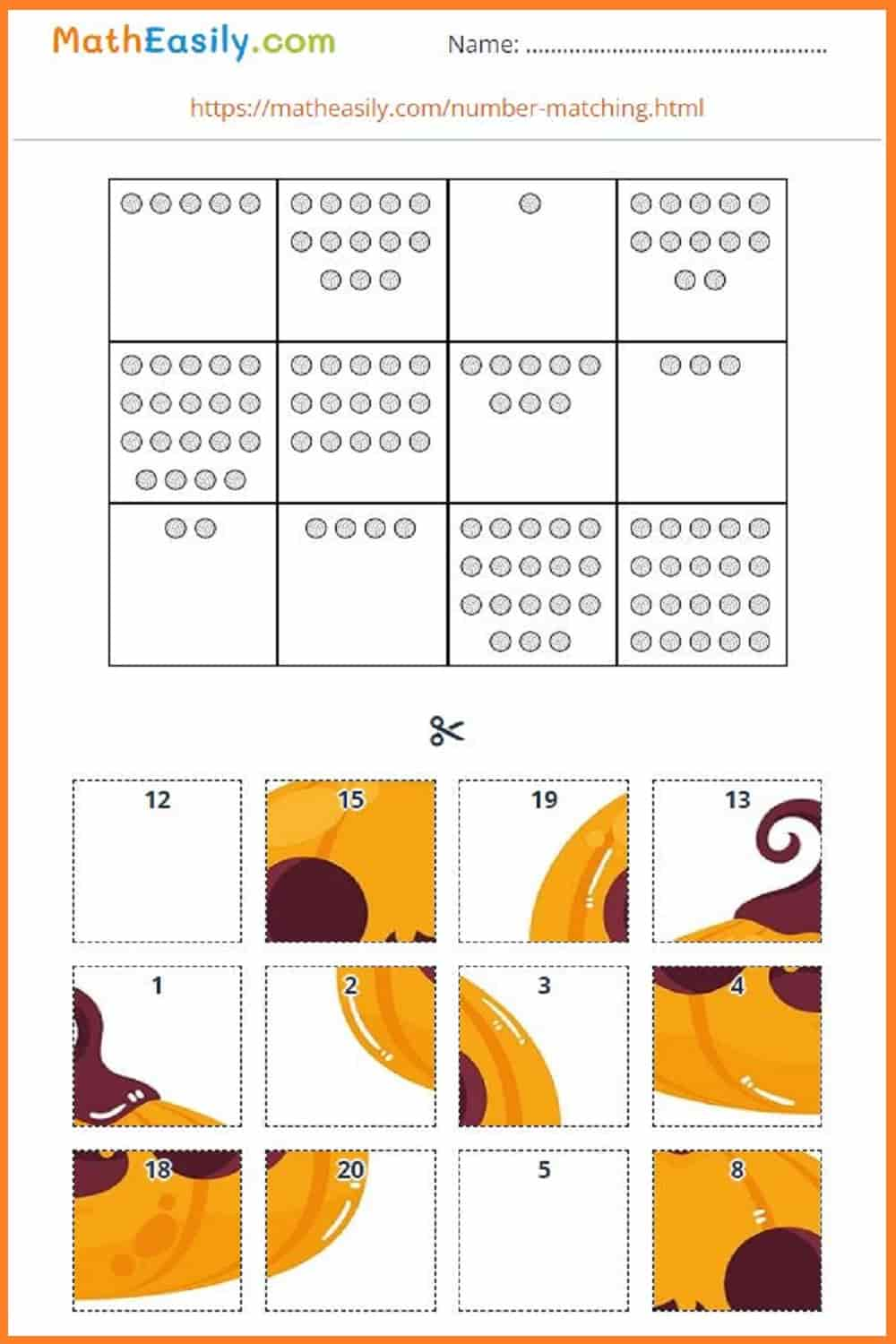Generate a new PDF

### Counting games

Generate Halloween counting worksheets 1-10.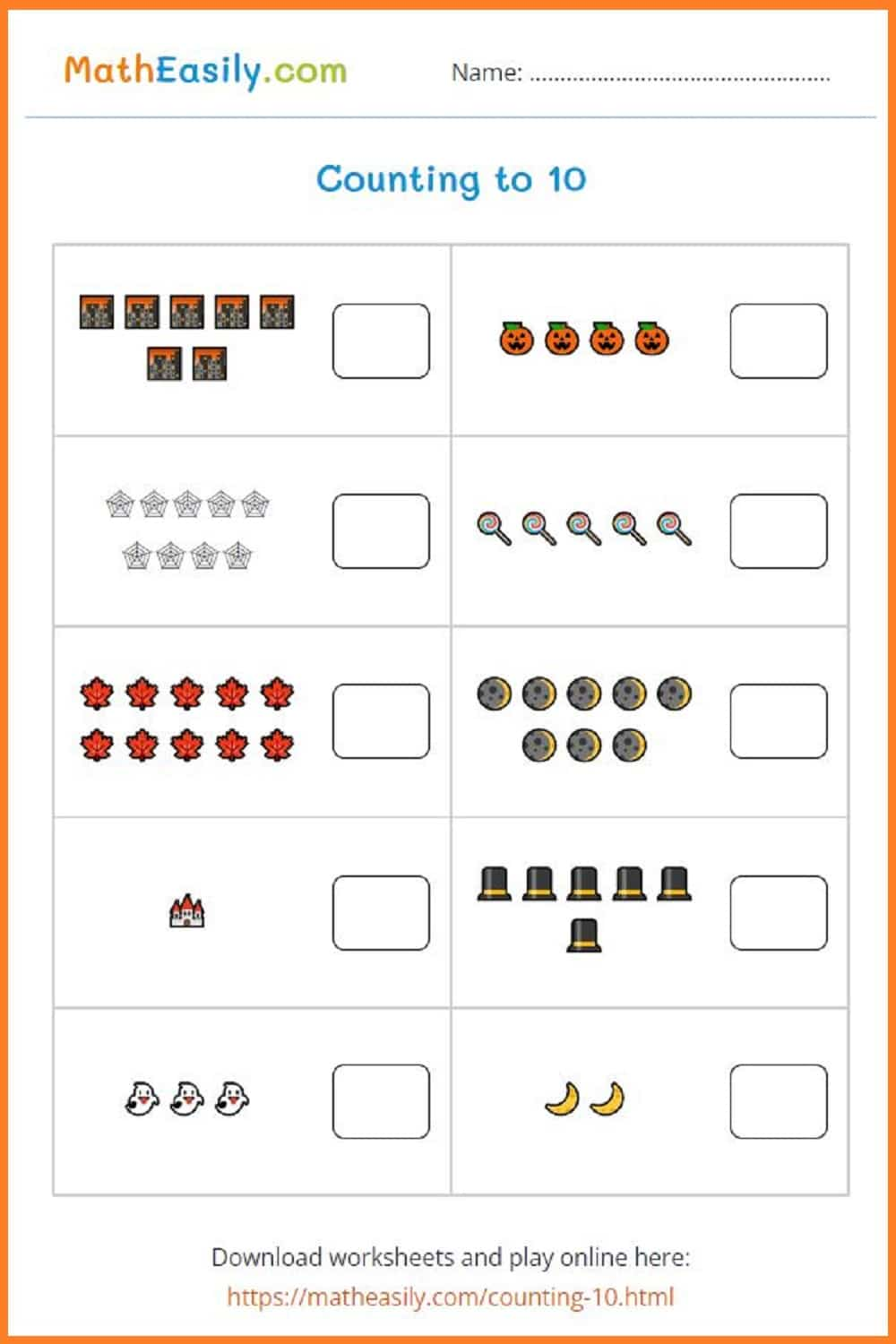Generate a new PDF

### Counting games to 20

Generate Halloween math worksheets: counting 1-20.Generate a new PDF

Generate math Halloween worksheets: addition to 10.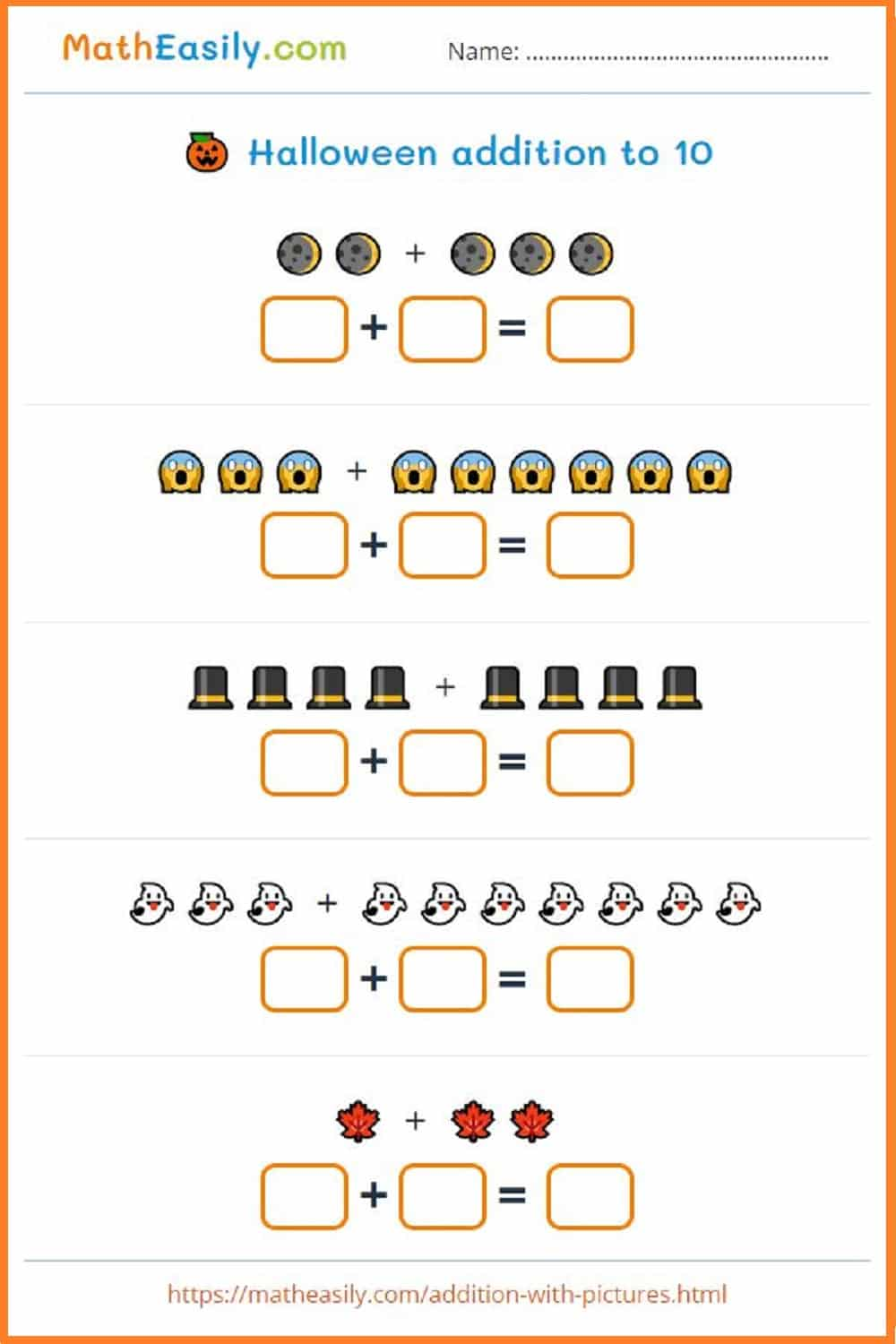Generate a new PDF

Generate Halloween math worksheets: addition to 10.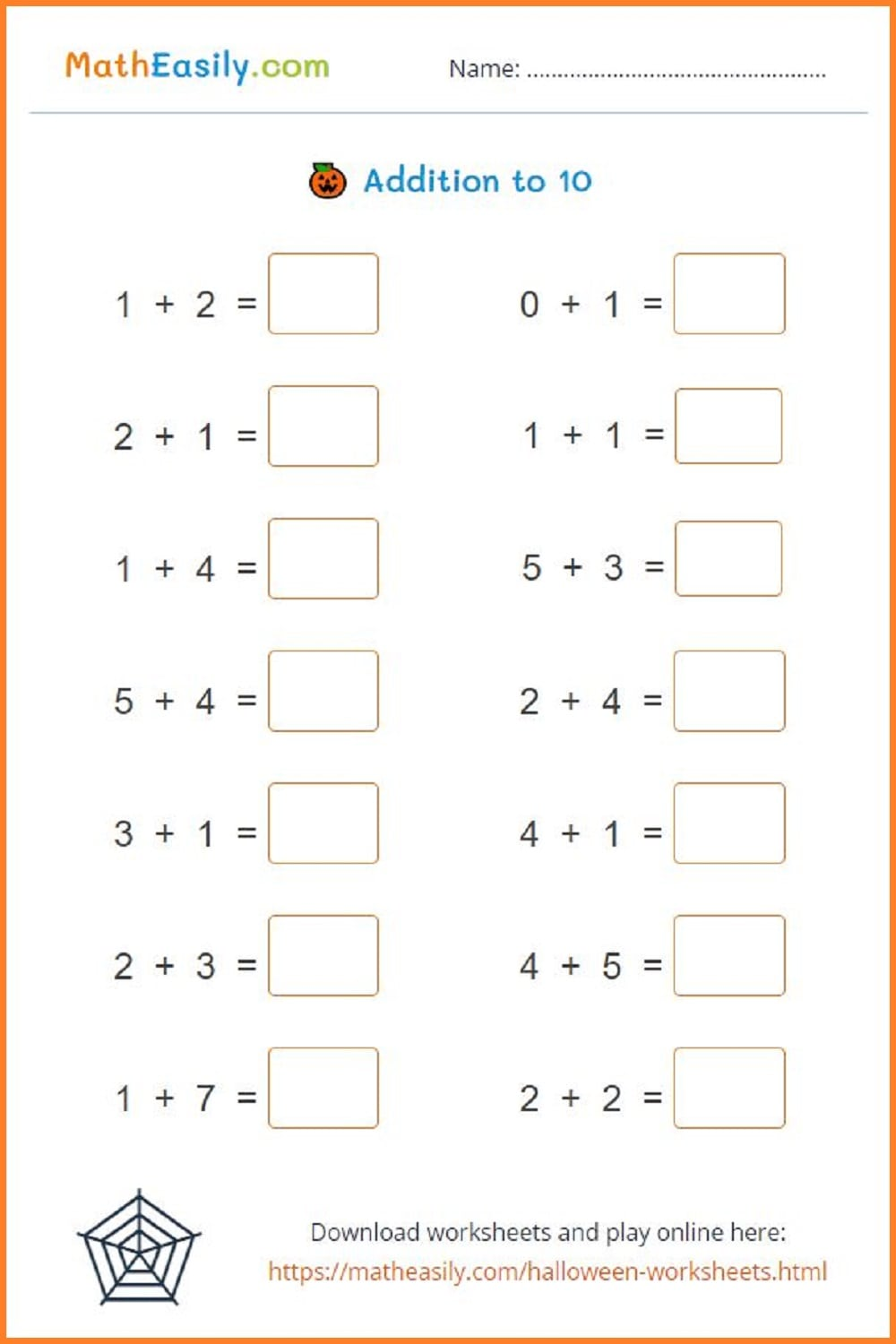Generate a new PDF

Generate math Halloween puzzles: addition to 20.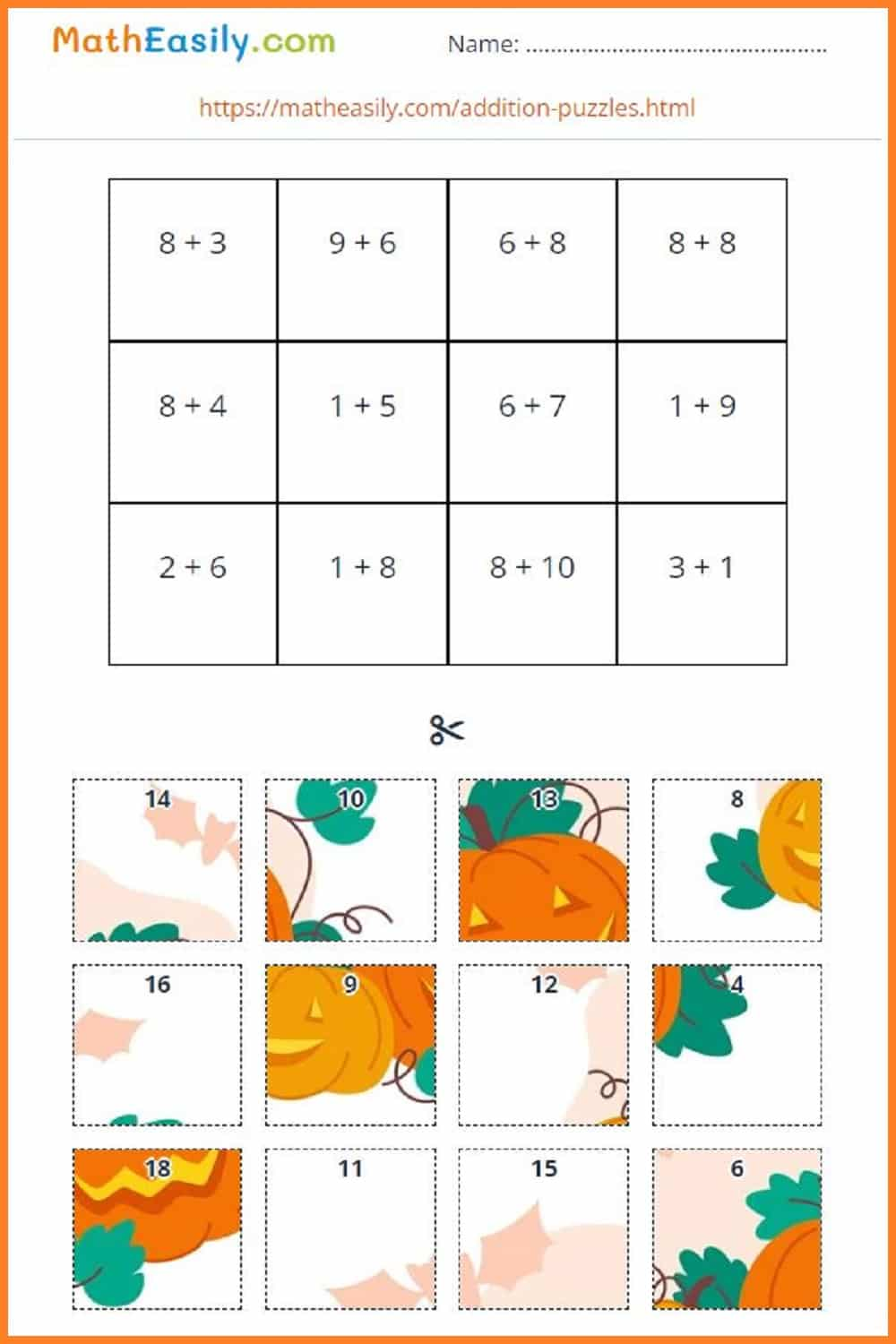Generate a new PDF

Generate math Halloween worksheets: addition to 20.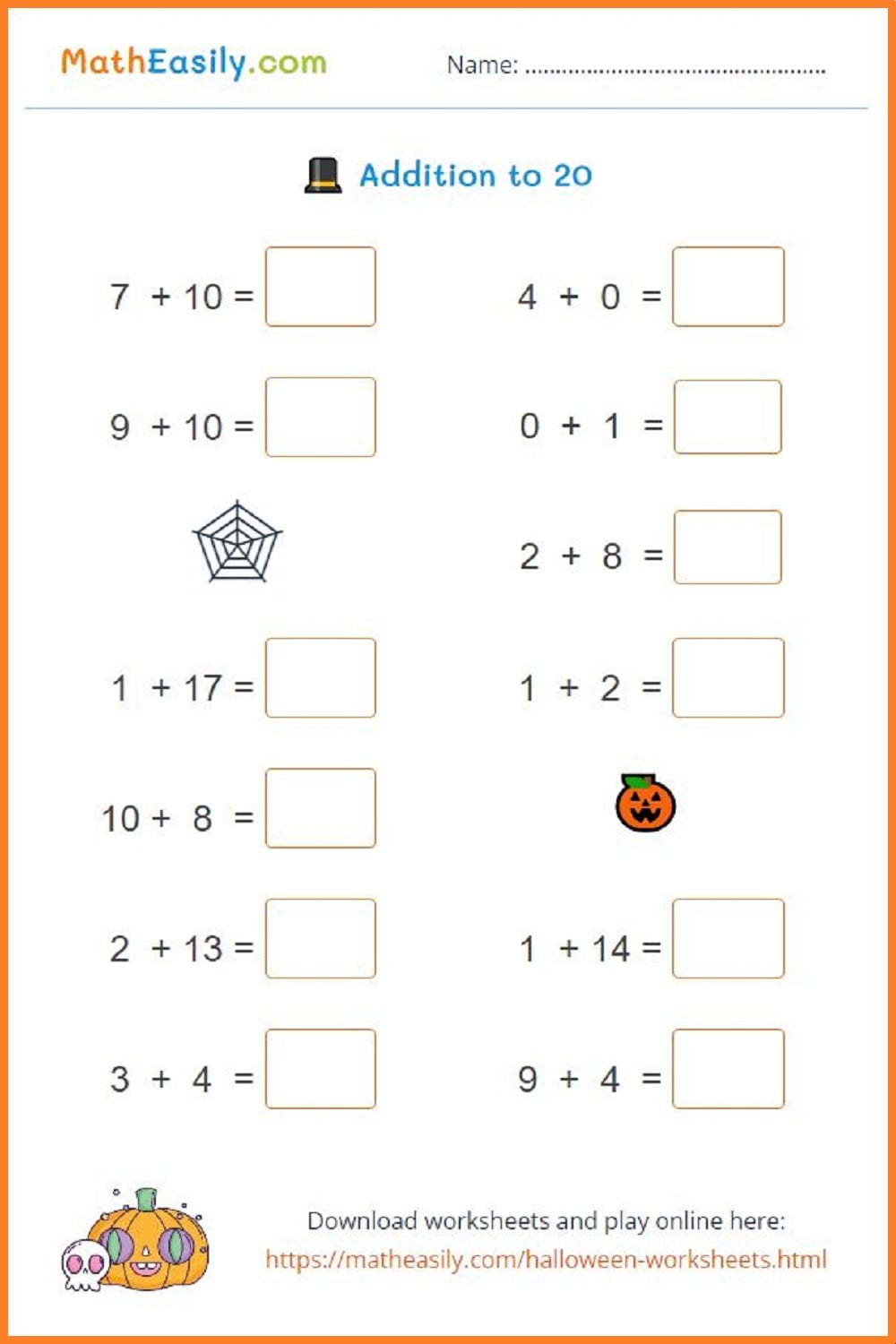Generate a new PDF

### Single digit subtraction

Subtraction with pictures within 10.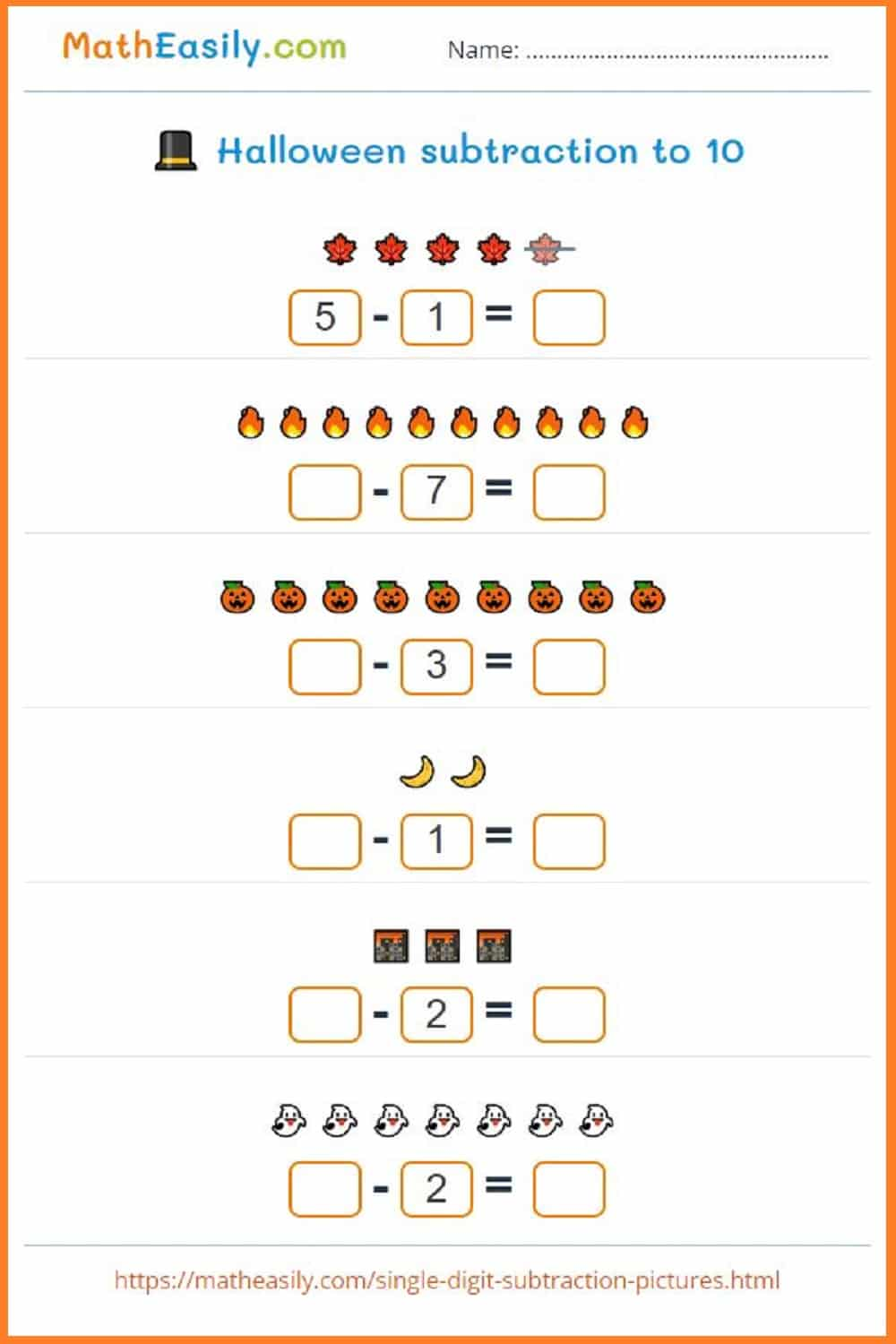Generate a new PDF

### Subtraction to 10

Halloween worksheets: subtraction within 10.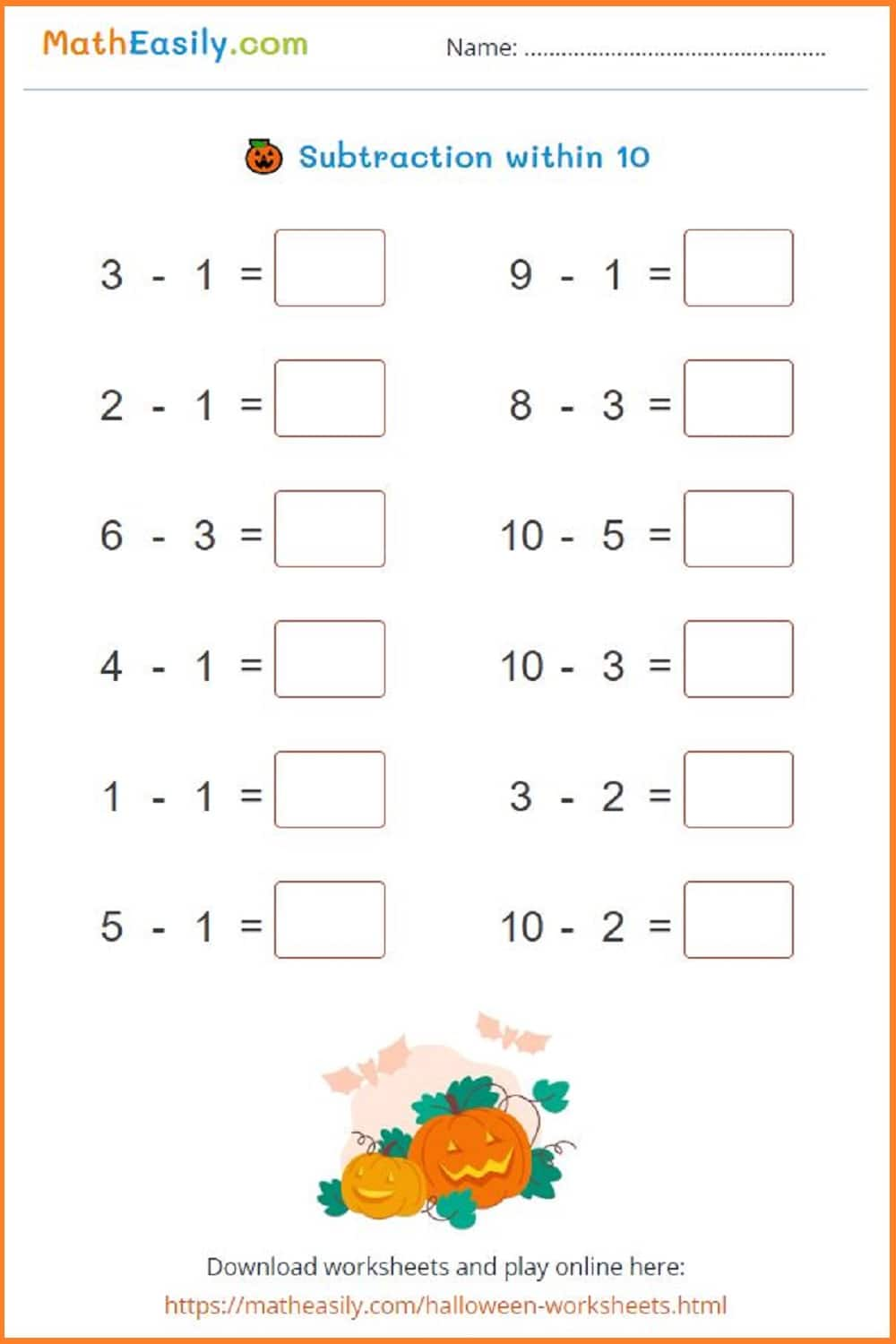Generate a new PDF

### Subtraction puzzles

Generate math Halloween puzzles: subtraction to 20.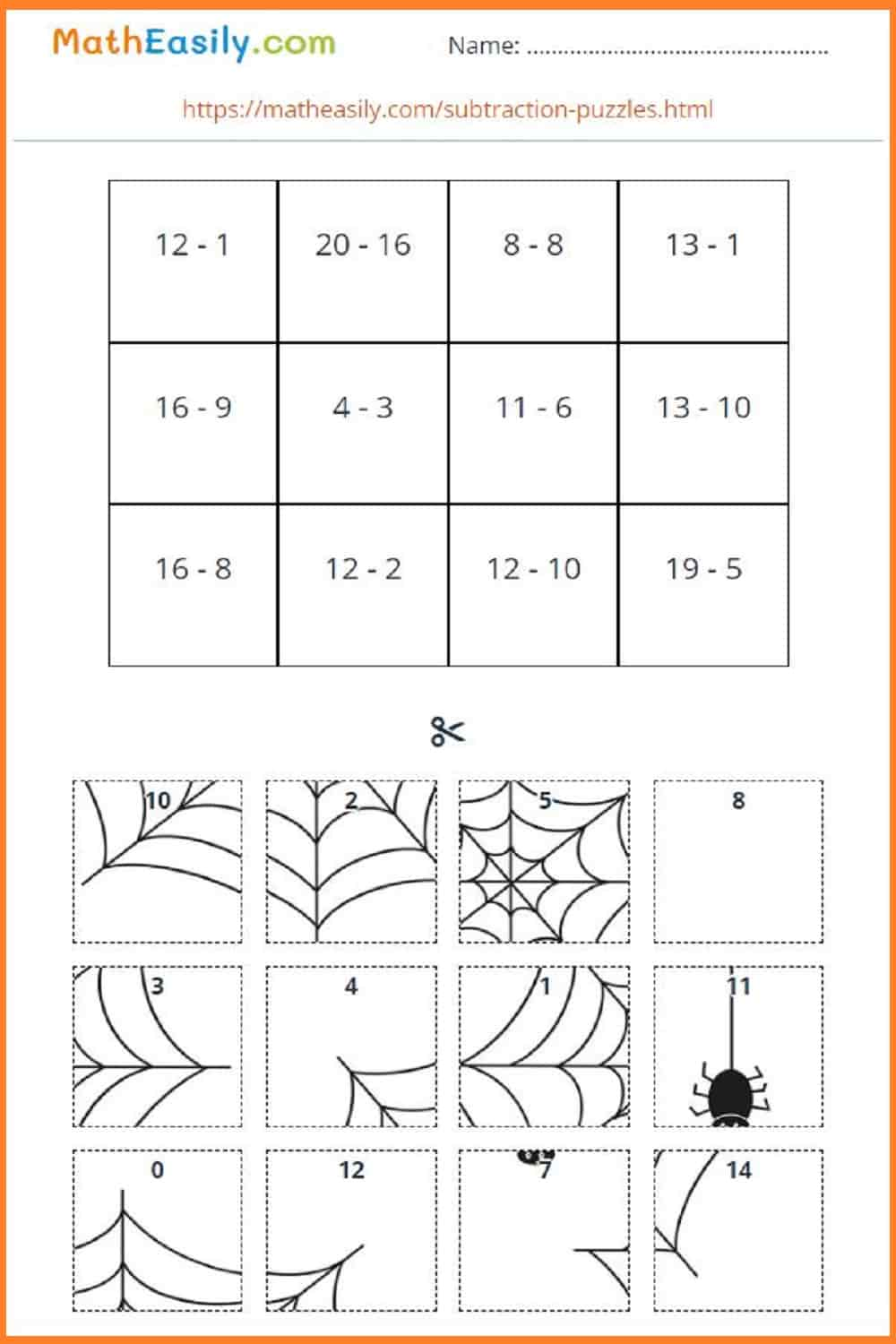Generate a new PDF

### Subtraction to 20

Halloween worksheets: subtraction within 20.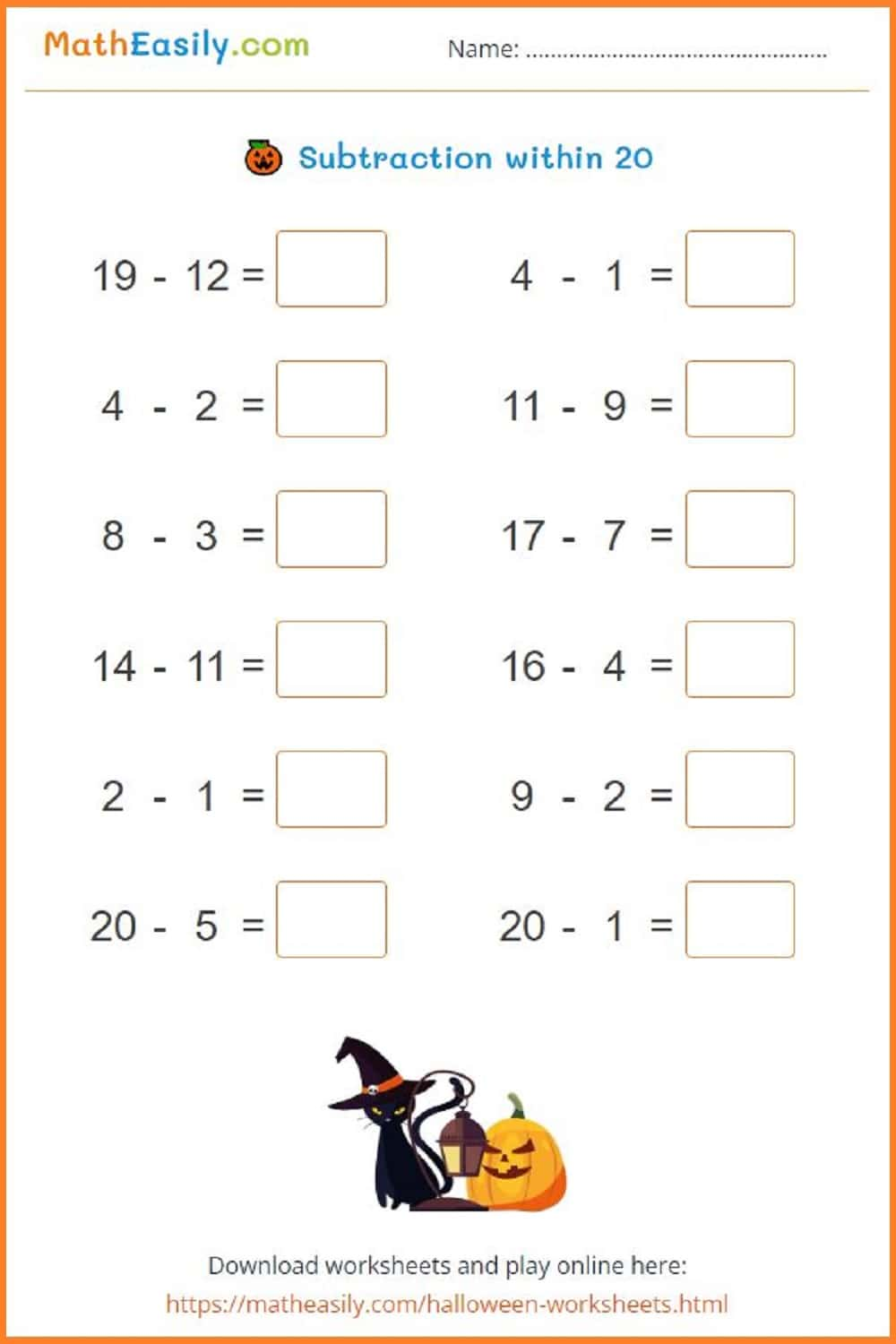Generate a new PDF

### Subtraction games

Halloween worksheets: subtraction within 30.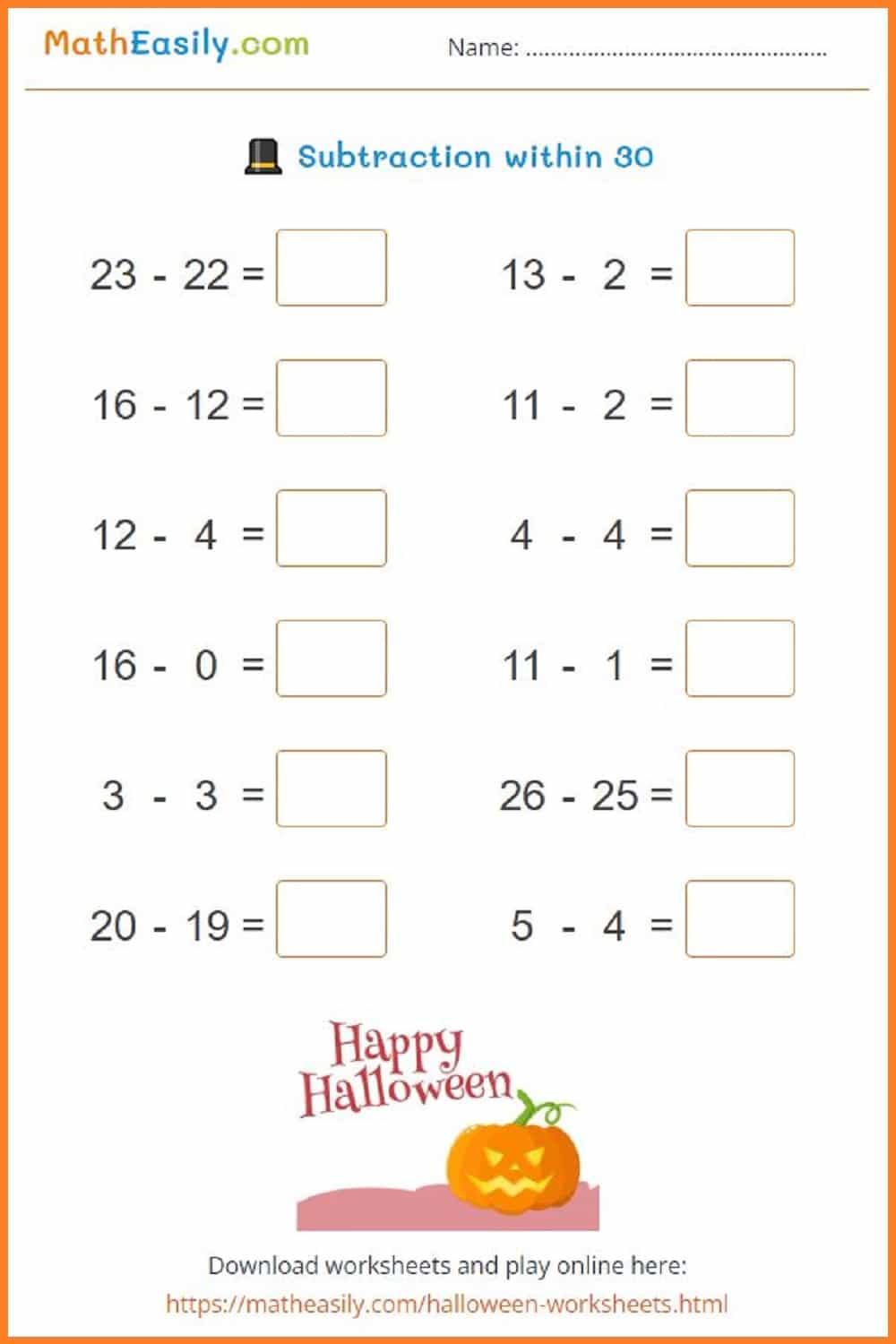Generate a new PDF

### Multiplication puzzles

Generate math Halloween multiplication puzzles in PDF.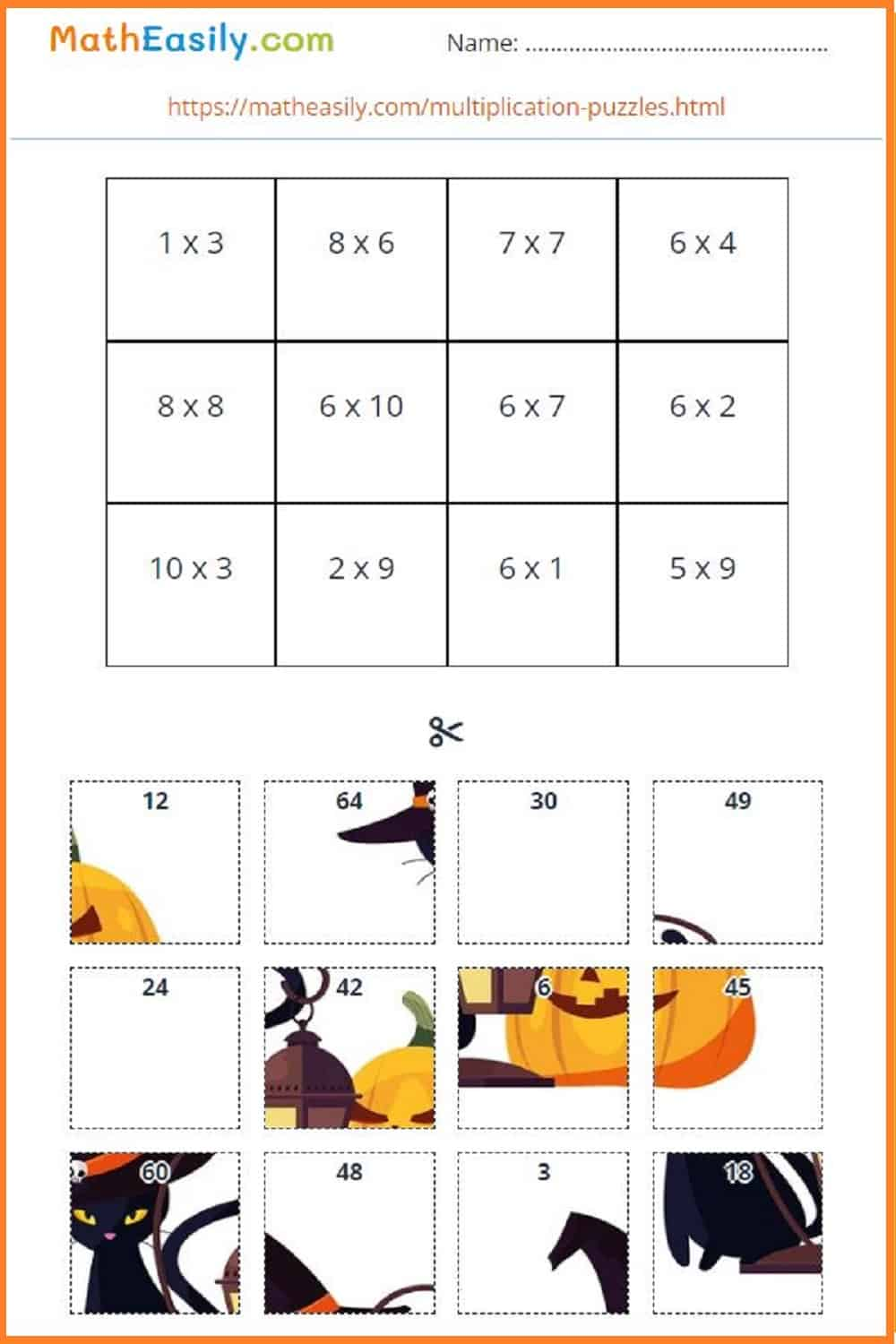Generate a new PDF

### Multiplication crossword

Generate multiplication Halloween worksheets.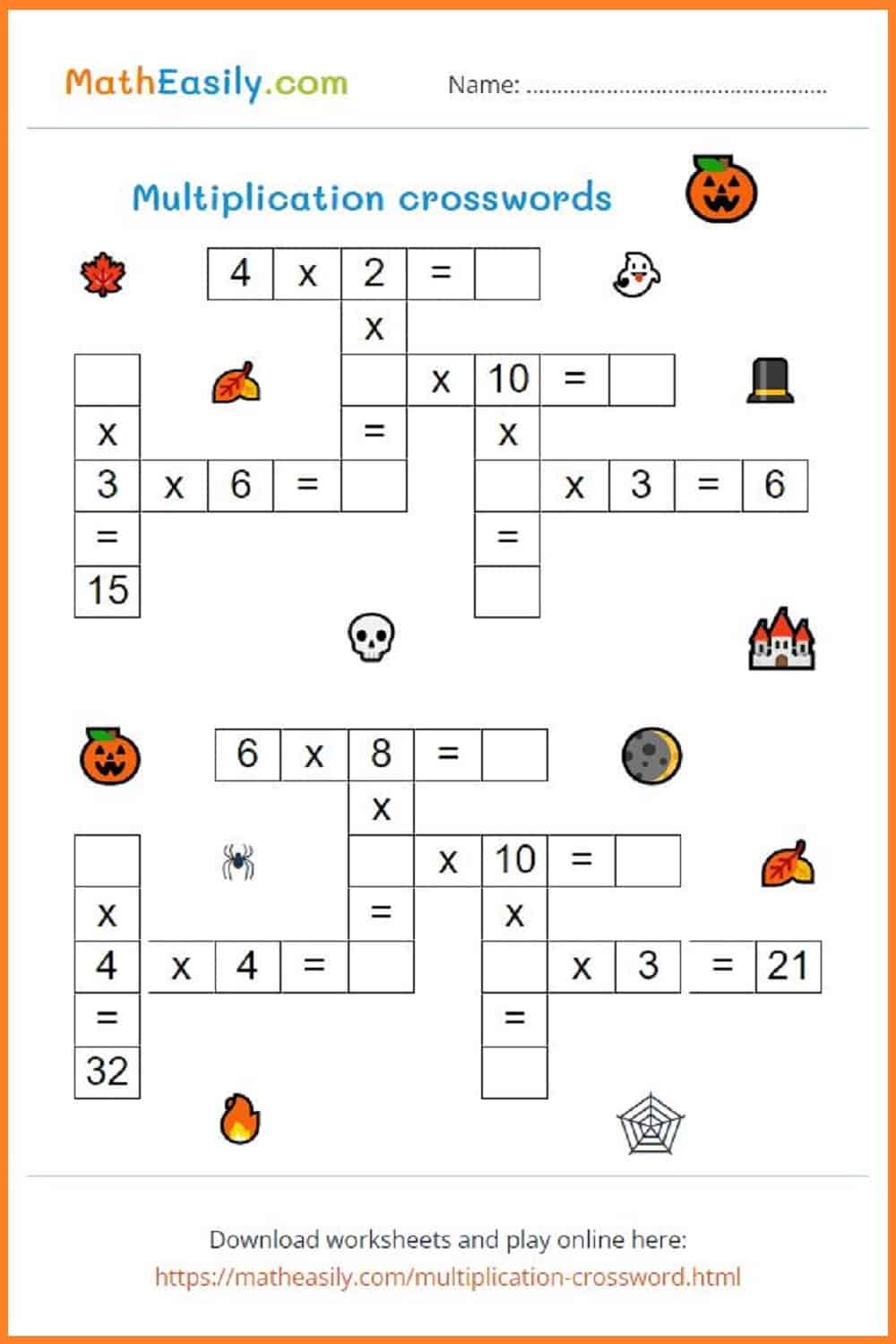Generate a new PDF

### Division puzzles

Generate math Christmas division puzzles in PDF.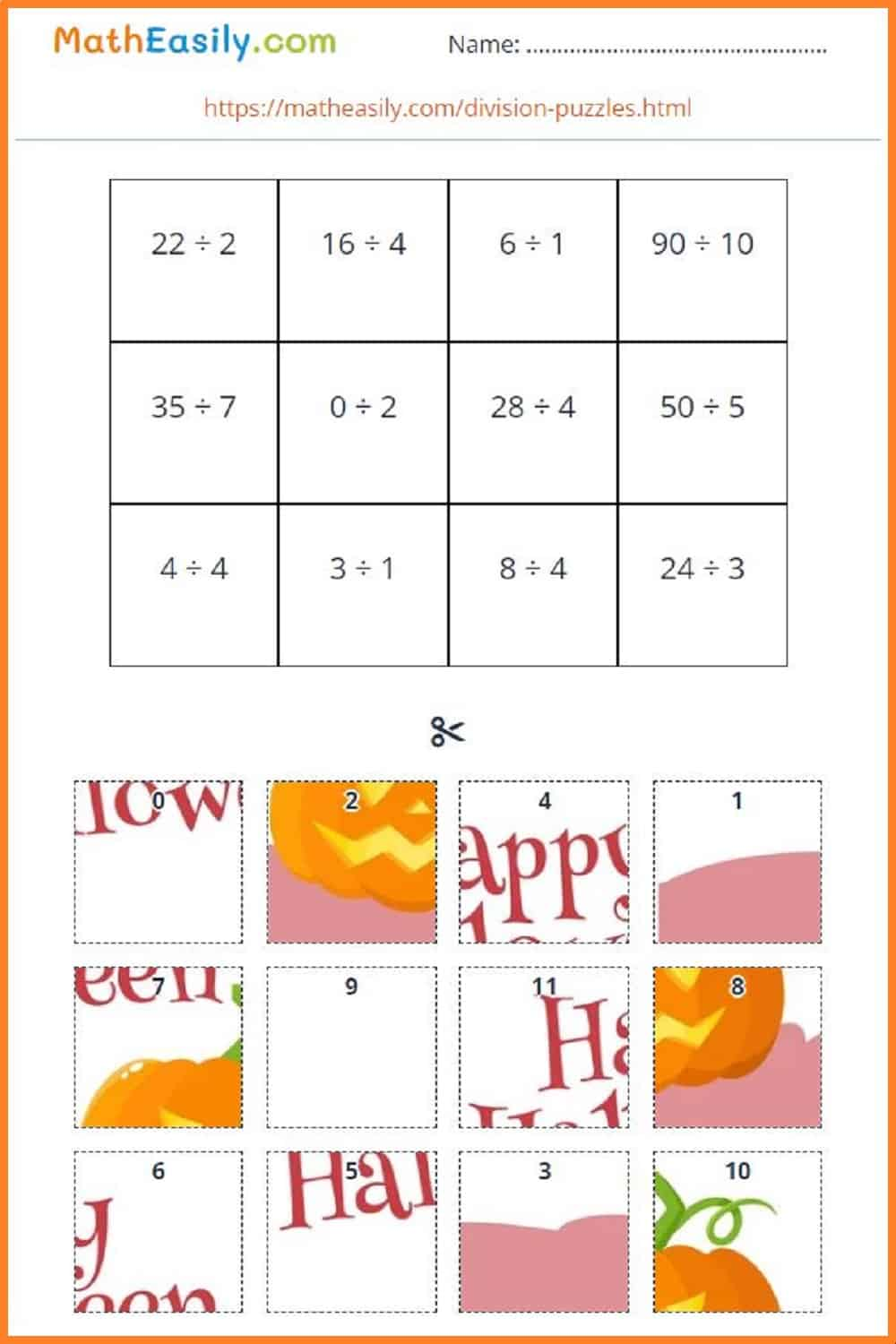Generate a new PDF

##### 100 Matchstick Puzzles in PDF

Printable math matchstick puzzles with answers. Move 1 stick to fix the equation.

US\$ 7.00

LOOK INSIDE

## 🎩 Fun Halloween maths activities

Below you will find a few Halloween maths activities from Pinterest. These colourful math Halloween worksheets will encourage children to practise math skills at this special time of the year.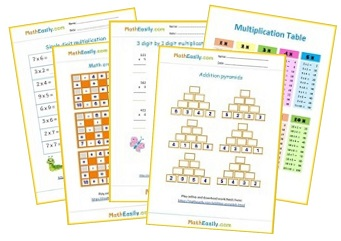Free Math Worksheets

## 🌒 Math Halloween worksheets PDF

Math is a key skill for kids to learn at any time of the year, and even Halloween is as good a time as any to get some practice in with your children.

Our free math Halloween worksheets are PDF documents which you can easily download. Just click on picture of the Halloween worksheet and generate a new PDF.

#### Other math worksheets

• Kindergarten Math Worksheets